# 1 ANCOVA model: Faculty political tolerances

A political scientist developed a questionnaire to determine political tolerance scores for a random sample of faculty members at her university. She wanted to compare mean scores adjusted for the age for each of the three categories: full professors (coded 1), associate professors (coded 2), and assistant professors (coded 3). The data are given below. Note the higher the score, the more tolerant the individual.

Below we will fit and interpret a model to assess the dependence of tolerance score on age and rank.

library(tidyverse)

#   save in the same folder as this Rmd file.

dat_tolerate <-
mutate(
# set 3="Asst" as baseline level
rank = factor(rank) %>% relevel(3)
, id = 1:n()
)
str(dat_tolerate)
tibble [30 x 4] (S3: spec_tbl_df/tbl_df/tbl/data.frame)
$score: num [1:30] 3.03 4.31 5.09 3.71 5.29 2.7 2.7 4.02 5.52 4.62 ...$ age  : num [1:30] 65 47 49 41 40 61 52 45 41 39 ...
$rank : Factor w/ 3 levels "3","1","2": 2 2 2 2 2 2 2 2 2 2 ...$ id   : int [1:30] 1 2 3 4 5 6 7 8 9 10 ...
- attr(*, "spec")=
.. cols(
..   score = col_double(),
..   age = col_double(),
..   rank = col_double()
.. )

## 1.1(0 p) Describe the plotted fitted regression lines

Below is a plot of tolerance against age, using rank as a plotting symbol. Describe how tolerance score depends on age within ranks.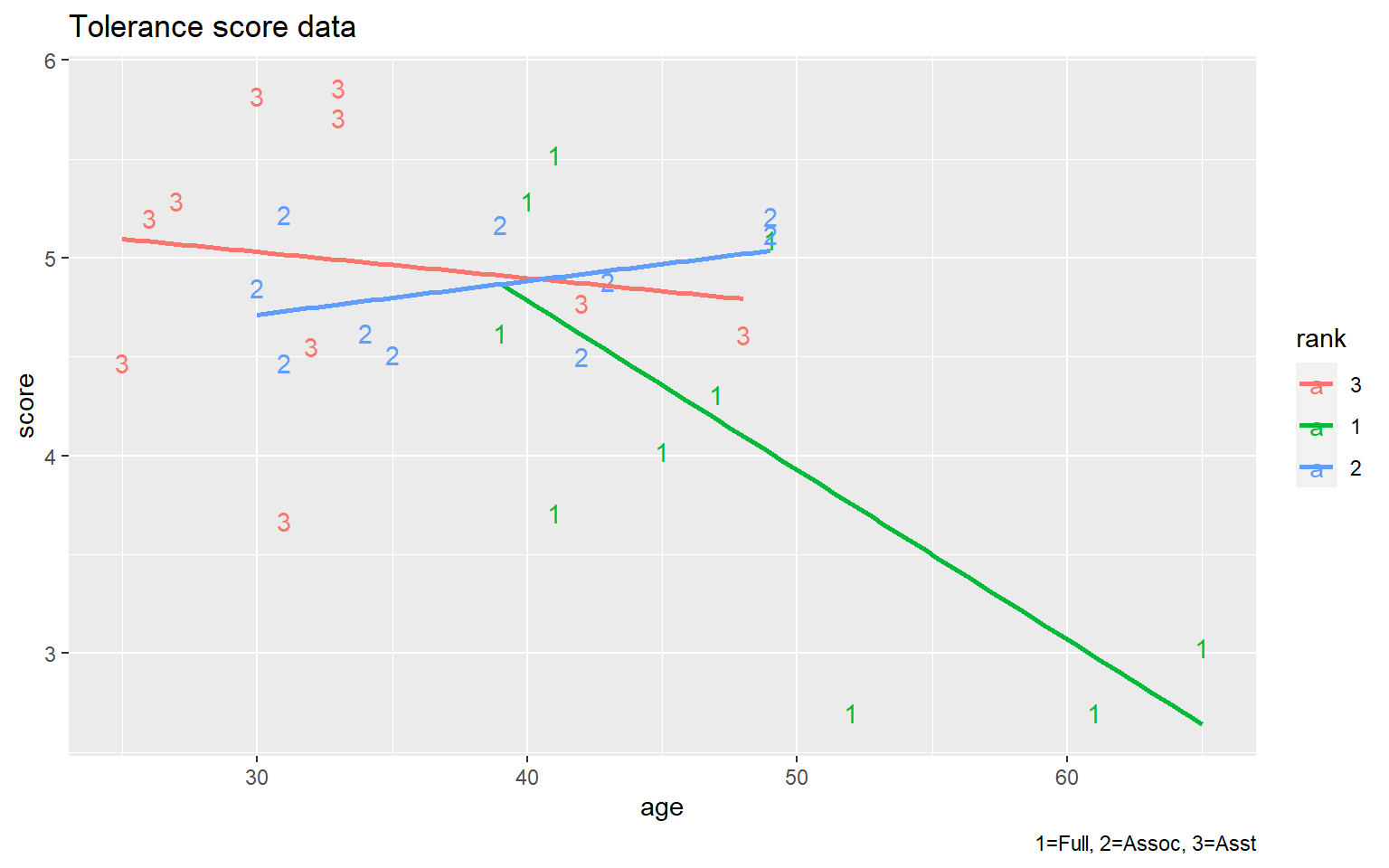### 1.1.1 Solution

The data plot suggests that tolerance decreases roughly linearly with age among the full professors (rank=1). The relationship between tolerance and age is much weaker (basically horizontal, no relationship) among the assistant professors (rank=3) and the associate professors (rank=2).

## 1.2(0 p) Write the full model equation with indicator variables.

(You did this last class.)

Create indicators for full and associate professors, so that assistant professors serve as the reference group. Write the full model, then the separate model for each rank using general notation.

### 1.2.1 Solution

We are interested in creating a multiple regression model that allows each rank to have its own regression line. There are three ranks, so two indicator variables are needed to uniquely identify each faculty member by rank. To have assistant professors serve as the reference group, let $$I(\textrm{rank}=1)=1$$ for full professors (rank=1) and $$I(\textrm{rank}=1)=0$$ otherwise, and set $$I(\textrm{rank}=2)=1$$ for associate professors (rank=2) and $$I(\textrm{rank}=2)=0$$ otherwise. Also define the two interaction or product effects: $$I(\textrm{rank}=1)\textrm{ age}$$ and $$I(\textrm{rank}=2)\textrm{ age}$$.

The model that allows separate slopes and intercepts for each rank is given by: $\textrm{score} = \beta_0 + \beta_1 I(\textrm{rank}=1) + \beta_2 I(\textrm{rank}=2) + \beta_3 \textrm{ age} + \beta_4 I(\textrm{rank}=1)\textrm{ age} + \beta_5 I(\textrm{rank}=2)\textrm{ age} + e.$ For later reference, the model will be expressed by considering the three faculty ranks separately. For assistant professors with rank = 3, we have $$I(\textrm{rank}=1)=I(\textrm{rank}=2)=0$$, so $\textrm{score} \ = \ \beta_0 + \beta_3 \textrm{ age} + e.$ For associates with rank=2, we have $$I(\textrm{rank}=1)=0$$ and $$I(\textrm{rank}=2)=1$$, which gives $\textrm{score} \ = \ \beta_0 + \beta_2(1) + \beta_3 \textrm{ age} + \beta_5 \textrm{ age} + e \ = \ (\beta_0 + \beta_2) + (\beta_3 + \beta_5) \textrm{ age} + e.$ Lastly, for full professors with rank=1, we have $$I(\textrm{rank}=2)=0$$ and $$I(\textrm{rank}=1)=1$$, so $\textrm{score} \ = \ \beta_0 + \beta_1(1) + \beta_3 \textrm{ age} + \beta_4 \textrm{ age} + e \ = \ (\beta_0 + \beta_1) + (\beta_3 + \beta_4) \textrm{ age} + e.$

The regression coefficients $$\beta_0$$ and $$\beta_3$$ are the intercept and slope for the assistant professor population regression line. The other parameters measure differences in intercepts and slopes across the three groups, using assistant professors as a baseline or reference group. In particular:

$$\beta_1 =$$ difference between the intercepts of the full and assistant professors population regression lines.

$$\beta_2 =$$ difference between the intercepts of the associate and assistant professors population regression lines.

$$\beta_4 =$$ difference between the slopes of the full and assistant professors population regression lines.

$$\beta_5 =$$ difference between the slopes of the associate and assistant professors population regression lines.

## 1.3(2 p) Test for equal slopes.

Starting with a model that allows each rank to have it’s own intercept and slope, test whether the slopes are equal. If the hypothesis of equal slopes is plausible, fit the model of equal slopes and test whether intercepts are equal.

lm_s_a_r_ar <-
lm(
score ~ age * rank
, data = dat_tolerate
)

# plot diagnostics
lm_diag_plots(lm_s_a_r_ar)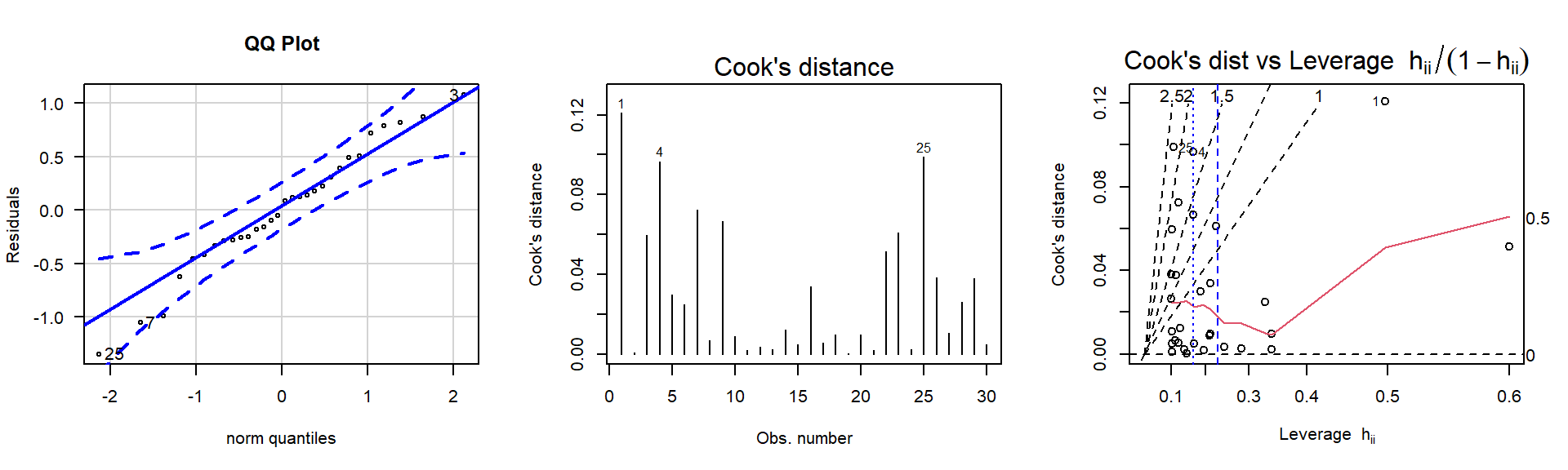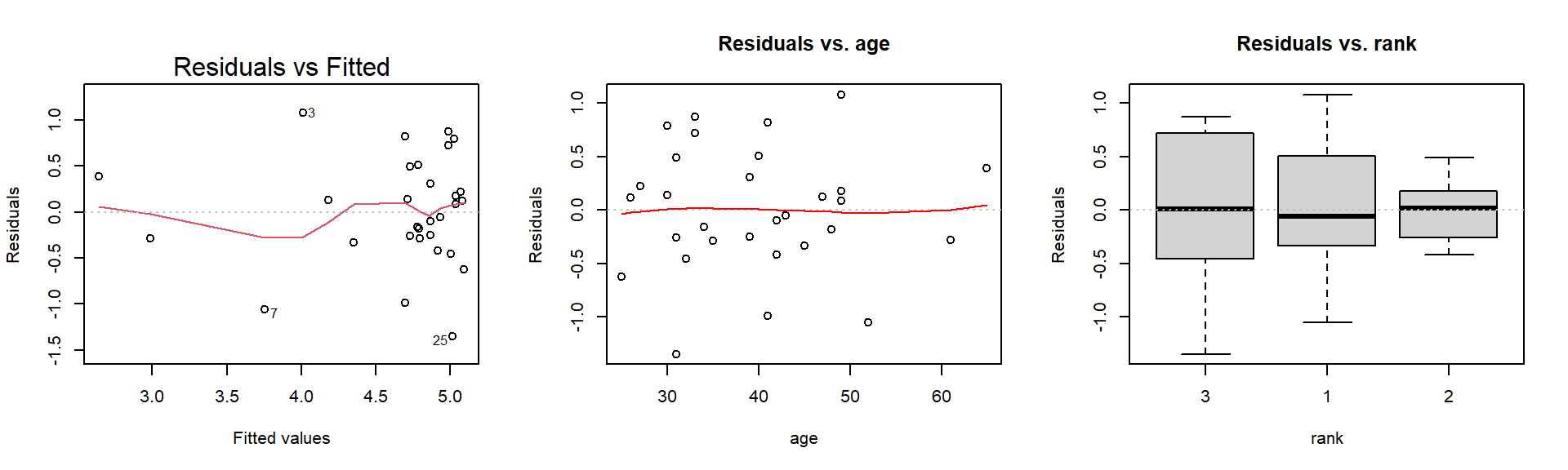Non-constant Variance Score Test
Variance formula: ~ fitted.values
Chisquare = 0.05251352, Df = 1, p = 0.81875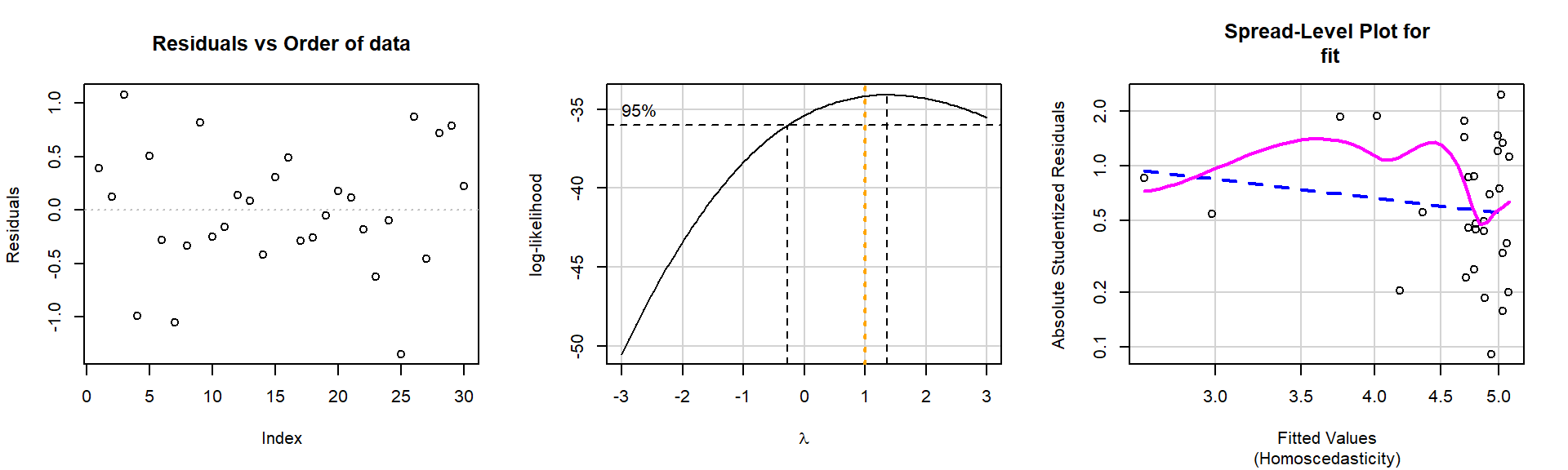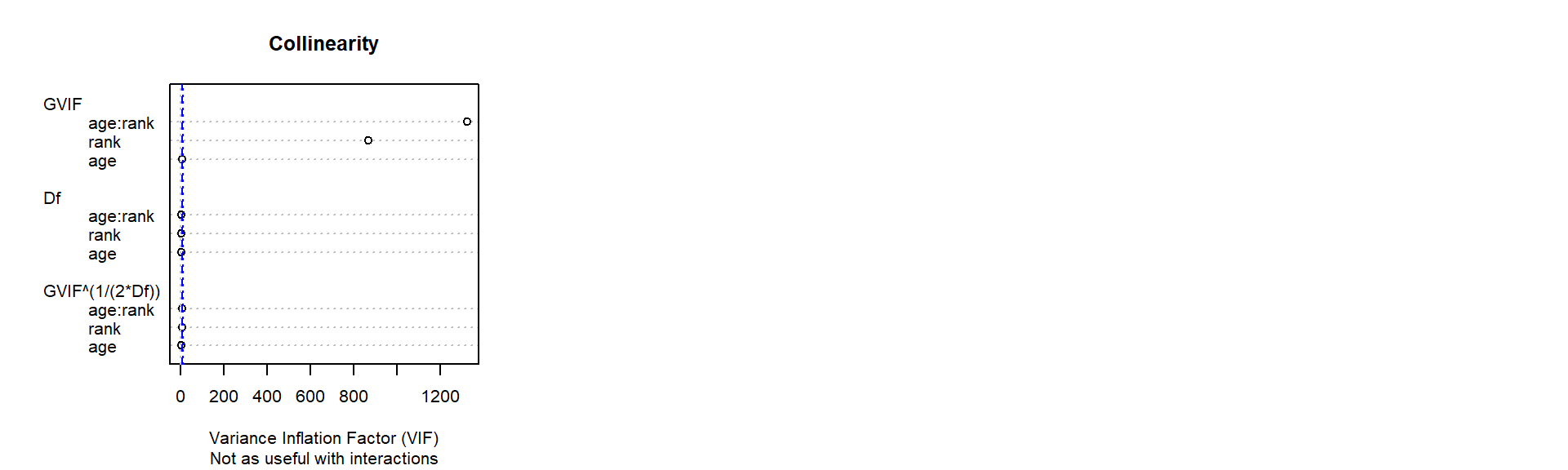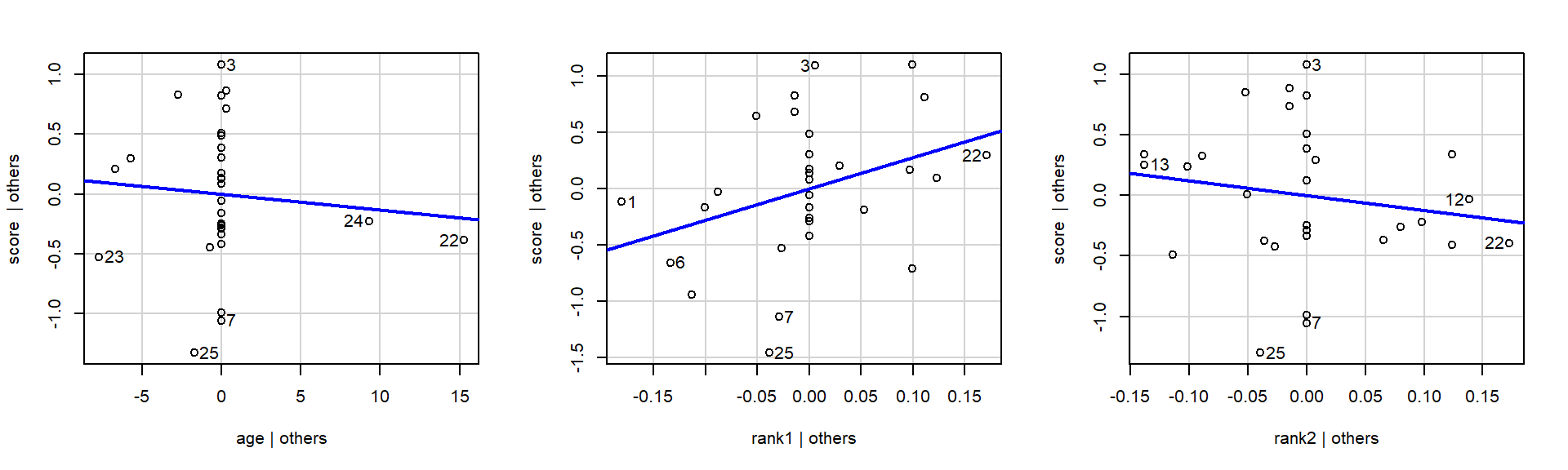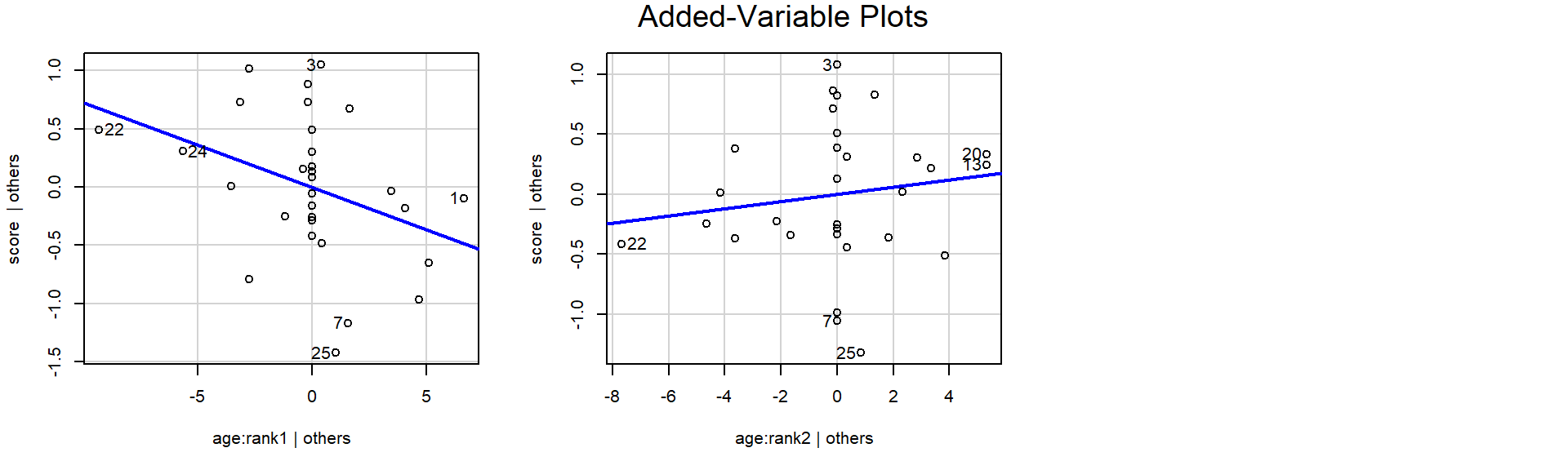Then, test the hypothesis of equal slopes.

library(car)
Anova(aov(lm_s_a_r_ar), type=3)
Anova Table (Type III tests)

Response: score
Sum Sq Df F value    Pr(>F)
(Intercept) 12.3528  1 30.3672 1.148e-05 ***
age          0.0817  1  0.2009   0.65802
rank         2.6243  2  3.2257   0.05744 .
age:rank     3.3610  2  4.1312   0.02872 *
Residuals    9.7628 24
---
Signif. codes:  0 '***' 0.001 '**' 0.01 '*' 0.05 '.' 0.1 ' ' 1

## 1.4(1 p) Reduce the model.

Given the tests in the previous part, reduce the model using backward selection.

2. If slopes are equal (not significantly for being different), then test for equal intercepts.
3. If intercepts are equal, test for any slope.
4. If slope is zero, then the grand mean intercept is the best model.

## 1.5(0 p) Write the fitted model equation.

Last class you wrote these model equations. Modify to your reduced model if necessary.

summary(lm_s_a_r_ar)

Call:
lm(formula = score ~ age * rank, data = dat_tolerate)

Residuals:
Min       1Q   Median       3Q      Max
-1.34746 -0.28793  0.01405  0.36653  1.07669

Coefficients:
Estimate Std. Error t value Pr(>|t|)
(Intercept)  5.42706    0.98483   5.511 1.15e-05 ***
age         -0.01321    0.02948  -0.448   0.6580
rank1        2.78490    1.51591   1.837   0.0786 .
rank2       -1.22343    1.50993  -0.810   0.4258
age:rank1   -0.07247    0.03779  -1.918   0.0671 .
age:rank2    0.03022    0.04165   0.726   0.4751
---
Signif. codes:  0 '***' 0.001 '**' 0.01 '*' 0.05 '.' 0.1 ' ' 1

Residual standard error: 0.6378 on 24 degrees of freedom
Multiple R-squared:  0.5112,    Adjusted R-squared:  0.4093
F-statistic:  5.02 on 5 and 24 DF,  p-value: 0.002748

### 1.5.1 Solution

Modify if your reduced model is different.

1: full professors $\widehat{\textrm{score}} = 5.427 + 2.785 + (-0.013 -0.072) \textrm{ age} = 8.212 - 0.085 \textrm{ age}$

2: associate professors $\widehat{\textrm{score}} = 5.427 - 1.223 + (-0.013 +0.030) \textrm{ age} = 4.204 + 0.017 \textrm{ age}$

3: assistant professors $\widehat{\textrm{score}} = 5.427 - 0.013 \textrm{ age}$

## 1.6(1 p) Aside: regression line estimation with interaction

(The question is at the bottom of this exposition.)

One feature to notice is that the observation 7 in the group of full professors appears to have an unusually low tolerance for his age (2.70 52 1). If you temporarily hold this observation out of the analysis, you still conclude that the population regression lines have different slopes.

# exclude observation 7 from tolerate7 dataset
dat_tolerate7 <-
dat_tolerate %>%
slice(-7)

lm7_s_a_r_ar <-
lm(
score ~ age * rank
, data = dat_tolerate7
)
library(car)
Anova(aov(lm7_s_a_r_ar), type=3)
Anova Table (Type III tests)

Response: score
Sum Sq Df F value    Pr(>F)
(Intercept) 12.3528  1 33.4564 6.821e-06 ***
age          0.0817  1  0.2213   0.64245
rank         2.3381  2  3.1662   0.06100 .
age:rank     2.8667  2  3.8821   0.03526 *
Residuals    8.4921 23
---
Signif. codes:  0 '***' 0.001 '**' 0.01 '*' 0.05 '.' 0.1 ' ' 1
summary(lm7_s_a_r_ar)

Call:
lm(formula = score ~ age * rank, data = dat_tolerate7)

Residuals:
Min       1Q   Median       3Q      Max
-1.34746 -0.31099  0.01162  0.30310  0.94978

Coefficients:
Estimate Std. Error t value Pr(>|t|)
(Intercept)  5.42706    0.93827   5.784 6.82e-06 ***
age         -0.01321    0.02808  -0.470   0.6425
rank1        2.58793    1.44812   1.787   0.0871 .
rank2       -1.22343    1.43853  -0.850   0.4038
age:rank1   -0.06586    0.03618  -1.821   0.0817 .
age:rank2    0.03022    0.03968   0.762   0.4540
---
Signif. codes:  0 '***' 0.001 '**' 0.01 '*' 0.05 '.' 0.1 ' ' 1

Residual standard error: 0.6076 on 23 degrees of freedom
Multiple R-squared:  0.4706,    Adjusted R-squared:  0.3555
F-statistic: 4.088 on 5 and 23 DF,  p-value: 0.0084

This observation has a fairly large impact on the estimated intercept and slope for the full professor regression line, but has no effect whatsoever on the estimated intercepts or slopes for the two other ranks. Why?

# full data set
coef(lm_s_a_r_ar)  %>% round(4)
(Intercept)         age       rank1       rank2   age:rank1   age:rank2
5.4271     -0.0132      2.7849     -1.2234     -0.0725      0.0302 
# without obs 7
coef(lm7_s_a_r_ar) %>% round(4)
(Intercept)         age       rank1       rank2   age:rank1   age:rank2
5.4271     -0.0132      2.5879     -1.2234     -0.0659      0.0302 

# 2 Additional analyses, possible directions

We’ll explore four possible sets of additional analyses that help us understand the relationships we found.

There are a number of possible directions here. We found earlier that there was an interaction, so there’s evidence for different slopes.

1. Use the Wald test to perform pairwise comparisons for the regression line slope between ranks.

2. Use the Wald test to perform pairwise comparisons for the regression line slope and intercept between ranks.

3. Observe that Full professors (rank = 1) are the only ones that have ages greater than 50, and those three observations are systematically different from scores for faculty not older than 50 – thus these three observations could be removed and inference could be limited to faculty from 25–50 years old.

4. Combine the junior faculty (assistant and associate: AA).

Other ideas are possible, but these are enough.

## 2.1(0 p) Direction 1: pairwise comparison of regression line slope between ranks

I’ll get you started using the Wald test to set up 1+ degree-of-freedom hypothesis tests.

Earlier we found that slopes are different. We will use the Wald test to perform comparisons of slopes between pairs of ranks.

We’ll discuss the linear algebra specification of these hypothesis test in class.

### 2.1.1 Solution

The tests below indicate that there’s an interaction because the slopes for Ranks 1 and 2 differ. Because we’re performing three tests, it is appropriate to compare these p-values to a significance level controlling the familywise Type-I error rate; the Bonferroni threshold is 0.05/3=0.01667.

# first, find the order of the coefficients
coef(lm_s_a_r_ar)
(Intercept)         age       rank1       rank2   age:rank1   age:rank2
5.42706473 -0.01321299  2.78490230 -1.22343359 -0.07247382  0.03022001 
library(aod) # for wald.test()

## H0: Slope of Rank 1 = Rank 3 (similar to summary table above)
mR <-
rbind(
c(0, 0, 0, 0, 1, 0)
) %>%
as.matrix()
vR <- c(0)

test_wald <-
wald.test(
b     = coef(lm_s_a_r_ar)
, Sigma = vcov(lm_s_a_r_ar)
, L     = mR
, H0    = vR
)
test_wald
Wald test:
----------

Chi-squared test:
X2 = 3.7, df = 1, P(> X2) = 0.055
## H0: Slope of Rank 2 = Rank 3 (similar to summary table above)
mR <-
rbind(
c(0, 0, 0, 0, 0, 1)
) %>%
as.matrix()
vR <- c(0)

test_wald <-
wald.test(
b     = coef(lm_s_a_r_ar)
, Sigma = vcov(lm_s_a_r_ar)
, L     = mR
, H0    = vR
)
test_wald
Wald test:
----------

Chi-squared test:
X2 = 0.53, df = 1, P(> X2) = 0.47
## H0: Slope of Rank 1 = Rank 2 (not in summary table above)
mR <-
rbind(
c(0, 0, 0, 0, 1, -1)
) %>%
as.matrix()
vR <- c(0)

test_wald <-
wald.test(
b     = coef(lm_s_a_r_ar)
, Sigma = vcov(lm_s_a_r_ar)
, L     = mR
, H0    = vR
)
test_wald
Wald test:
----------

Chi-squared test:
X2 = 7.4, df = 1, P(> X2) = 0.0065

## 2.2(2 p) Direction 2: pairwise comparison of regression lines (slope and intercept) between ranks

To test whether the regression line is different between ranks, in the null hypothesis $$H_0$$ we need to set both the slope and the intercept equal between a selected pair of ranks.

Here’s the first example:

# first, find the order of the coefficients
coef(lm_s_a_r_ar)
(Intercept)         age       rank1       rank2   age:rank1   age:rank2
5.42706473 -0.01321299  2.78490230 -1.22343359 -0.07247382  0.03022001 
library(aod) # for wald.test()

## H0: Line of Rank 1 = Rank 3
mR <-
rbind(
c(0, 0, 1, 0, 0, 0)
, c(0, 0, 0, 0, 1, 0)
) %>%
as.matrix()
vR <- c(0, 0)

test_wald <-
wald.test(
b     = coef(lm_s_a_r_ar)
, Sigma = vcov(lm_s_a_r_ar)
, L     = mR
, H0    = vR
)
test_wald
Wald test:
----------

Chi-squared test:
X2 = 3.7, df = 2, P(> X2) = 0.16

## 2.3(1 p) Direction 3: exclude ages $$> 50$$ and reanalyze

Drop observations with age > 50 and refit the model. Remember to check model assumptions, then do backward selection (manually), then check the final model assumptions.

## 2.4(3 p) Direction 4: Combine the junior faculty (asst and assoc)

Create a new factor variable rankaa that combines ranks 2 and 3 as value 0, but has rank 1 still value 1.

dat_tolerate <-
dat_tolerate %>%
mutate(
# indicator for Full vs (Assist & Assoc)
rankaa =
case_when(
rank %in% c(2, 3) ~ 0     # Assist & Assoc
, rank %in% c(1   ) ~ 1     # Full
)
, rankaa = factor(rankaa)
, rankaa = relevel(rankaa, "0")
)

Note that in Direction 2 above we tested whether the assistants and the associates have the same population regression line and found they were not statistically different. We had performed a simultaneous hypothesis test, same as below. (Note that this is an alternate way to do the simultaneous test when we are testing that the coefficients are equal to zero (using Terms = c(4, 6)); we did this differently above because I wanted to show the more general way of comparing whether coefficients were also equal to each other or possibly equal to a value different from zero).

coef(lm_s_a_r_ar)
(Intercept)         age       rank1       rank2   age:rank1   age:rank2
5.42706473 -0.01321299  2.78490230 -1.22343359 -0.07247382  0.03022001 
library(aod) # for wald.test()
# Typically, we are interested in testing whether individual parameters or
#   set of parameters are all simultaneously equal to 0s
# However, any null hypothesis values can be included in the vector coef.test.values.

coef_test_values <-
rep(0, length(coef(lm_s_a_r_ar)))

library(aod) # for wald.test()
test_wald <-
wald.test(
b     = coef(lm_s_a_r_ar) - coef_test_values
, Sigma = vcov(lm_s_a_r_ar)
, Terms = c(4, 6)
)
test_wald
Wald test:
----------

Chi-squared test:
X2 = 0.77, df = 2, P(> X2) = 0.68

The p-value for this test is approximately 0.7, which suggests that the population regression lines for these two groups are not significantly different.

At this point I would refit the model, omitting the $$I(\textrm{rank}=2)$$ and $$I(\textrm{rank}=2)\textrm{ age}$$ effects. $\textrm{score} = \beta_0 + \beta_1 I(\textrm{rank}=1) + \beta_3 \textrm{ age} + \beta_4 I(\textrm{rank}=1)\textrm{ age} + e.$ This model produces two distinct regression lines, one for the full professors and one for the combined assistants and associates.

Do this.

Using the combined AA rank data, do the following and interpret each result:

1. plot the data
2. fit the full interaction model, reduce if possible
3. write out the separate model equations for the Full and AA ranks
4. check model assumptions
5. reduce the model (if appropriate) and recheck assumptions